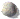Machine Learning: (I have done NO research but keen on learning)

# Research TopicsScheduling: competitive analysis, algorithms, lower bounds, resource augmentationVarying Degrees of Parallelizability: parallel, sequentialPower ManagementBroadcast SchedulingTCPLower BoundsBraching ProgramsCommunication ComplexityCake CuttingGreedy and Dynamic Programming AlgorithmsEmbedding DistortionTime-Space Trade-off Lower Bounds for st-Connectivity on JAG ModelsComplexity ClassesCircuit DepthComparisons Between Models of Parallel ComputationRandomnessJob DAGHandling DataData BasesData Transmission

## Machine Learning

Computers can now drive cars and find cancer in x-rays. For better or worse, this will change the world (and the job market). Strangely designing these algorithms is not done by telling the computer what to do or even by understanding what the computer does. The computers learn themselves from lots and lots of data and lots of trial and error. This learning process is more analogous to how brains evolved over billions of years of learning. The machine itself is a neural network which models both the brain and silicon and-or-not circuits, both of which are great for computing. The only difference with neural networks is that what they compute is determined by weights and small changes in these weights give you small changes in the result of the computation. The process for finding an optimal setting of these weights is analogous to finding the bottom of a valley. "Gradient Decent" achieves this by using the local slope of the hill (derivatives) to direct the travel down the hill, i.e. small changes to the weights. There is some theory. If a machine is found that gives the correct answers on the randomly chosen training data without simply memorizing, then we can prove that with high probability this same machine will also work well on never seen before instances.

Second level of Machine Learning (These topics are likely 30 min each)
- Generalizing from Training Data: We can prove that if we successfully train on enough randomly choose training data, then the produced machine generalities well to random examples never seen before.
- Reinforcement Learning & Markoff Chains: The basic theory needed to learn to solve some complex multi-step task.
- Dimension Reduction & Maximum Likelihood: How to compress your data while retaining the key features.
- Convolutional & Recurrent Networks: Used for learning images and sound that are invariant over location and time.
- Generative Adversarial Networks: Used for understanding and producing a random data item.
- Back Propagation: We could learn how to use matrix multiplication to learn the slope to your error space.
- Bayesian Inference: Given the probability of a symptom given a disease, we can compute the probability of a disease given a symptom.
- Decision Trees & Clustering: The basics.

## Scheduling Problems

Most broadly, this area of research considers the scheduling to a steady stream of incoming jobs, a shared resource such as processing time, battery power, or internet bandwidth. The problem is to determine how to best allocate and reallocate these resources to the jobs as they arrive and complete in a way that minimizes some global quality of service measure. One difficulty is the lack of knowledge that the scheduler has. The scheduler is said to be on-line if it does not know what jobs are going to arrive in the future, non-clairvoyant if it does not know the properties of the jobs currently in the system, and distributed if decisions are not made centrally. A second difficulty is that even with this information, most variants of the problem are NP-complete. The problem is to find in real time a schedule that is almost as good as the optimal schedule when you do not have complete information. We provide some answers to this question using the resource augmentation competitive analysis framework. This involves proving that given any input sequence, the algorithm, when given a constant times more of resource, performs within a constant times the efficiency as the all knowing all powerful optimal algorithm.

## Lower Bounds

For both practical and theoretical reasons, we would like to know the minimum amount of time (or space) needed to solve a given computational problem on an input of size n. An upper bound provides an algorithm that achieves some time bound. A lower bound proves that no algorithm correctly solves the problem faster no matter how clever. Doing this on general models of computation (eg. in JAVA or a Turing Machine) is beyond our reach. For this reason, researchers often prove lower bounds on weaker modes of computation.

## Handling Data

(These were two side papers. I don't supervise people in data bases)

.

.

.

.

.

.

.

.

.

.

.

.

.

.

.

.

.

.

.

.

.

.

.

.